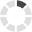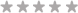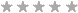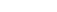### Site InformationLoading... Please wait...
• My Account

# Question 1 In a large city a certain (unknown) proportion of adults believe that global warming is a liberal hoax designed to hurt oil companies.

\$10.00Quantity:## Product Description

Question 1

In a large city a certain (unknown) proportion of adults believe that global warming is a liberal hoax designed to hurt oil companies.  You plan to take a survey of n adults to “estimate” this proportion. You want to be able to say that your estimate is within three percentage points (i.e., within 0.03) of the true proportion, with 0.95 probability.  What is the smallest sample size that guarantees that you would obtain a satisfactory estimate (an estimate that’s within 0.03 of the true proportion, with probability 0.95)? Provide an answer using an approximation based on the Central Limit Theorem.

 1068
 4269
 752
 2135

Flag this QuestionQuestion 2

In the population, “intelligence quotient” scores (IQ’s) are approximately normally distributed with a mean of 100 and a standard deviation of 15.  Suppose we plan to obtain IQ scores of a random sample of n = 30 individuals, and then we’ll compute the sample mean and sample standard deviation.

Find the probability that the sample mean will be larger than 105.

 0.20449
 0.00126
 0.36944
 0.03395

Flag this QuestionQuestion 3

In the population, “intelligence quotient” scores (IQ’s) are approximately normally distributed with a mean of 100 and a standard deviation of 15.  Suppose we plan to obtain IQ scores of a random sample of n = 30 individuals, and then we’ll compute the sample mean and sample standard deviation.

Find the values a and b (where a < b) such that the sample standard deviation is between a and b with probability 0.8, and the probability that the sample standard deviation is larger than b is 0.1.

 a = 13.205, b = 16.512
 a = 12.385, b = 17.415
 a = 11.721, b = 18.171
 a = 12.643, b = 17.673

Flag this QuestionQuestion 4

In the population, “intelligence quotient” scores (IQ’s) are approximately normally distributed with a mean of 100 and a standard deviation of 15.  Suppose we plan to obtain IQ scores of a random sample of n = 30 individuals, and then we’ll compute the sample mean and sample standard deviation.

Suppose you were unsure of the value of the population standard deviation, and so you plan to use only the sample standard deviation.  Find the exact probability that the sample mean will be within one-third of onesample standard deviation of the population mean.

 0.9611
 0.9218
 0.9609
 0.9221

Flag this QuestionQuestion 5

In the population, “intelligence quotient” scores (IQ’s) are approximately normally distributed with a mean of 100 and a standard deviation of 15. Suppose we plan to obtain IQ scores of a random sample of n = 30 individuals, and then we’ll compute the sample mean and sample standard deviation.

What would your answer to the question (4) be if instead you use an approximation based on large sample theory (i.e., based on the Central Limit Theorem)?

 0.9274
 0.9321
 0.9365
 0.9218

## Product Reviews

1. #### Worked for eco329Posted by on 18th Apr 2017

## Customers also viewed

• \$10.00• \$15.00• \$15.00• \$15.00• \$15.00## Related Products

Click the button below to add the Question 1 In a large city a certain (unknown) proportion of adults believe that global warming is a liberal hoax designed to hurt oil companies. to your wish list.